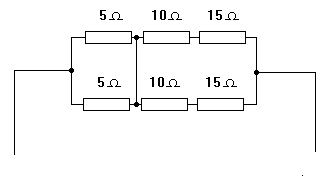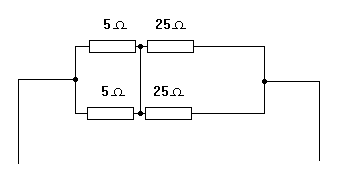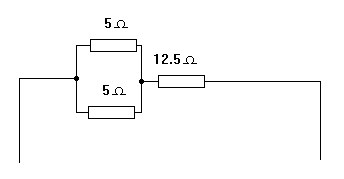Home > Electronics Tutorials > Direct Current (DC) Theory > Resistor Networks Tutorial

Direct Current (DC) Theory - Electronics Teaching

# Resistor Networks Tutorial

See the pages on series and parallel resistors before reading this one.In the diagram we have two sets of 10 ohms in series with 15 ohms.

These can be replaced by two 25 ohms as shown in the next diagram.The two 25 ohms are in parallel and can be replaced by 12.5 ohms.

See the next diagram.The two 5 ohms are in parallel so can be simplified to 2.5 ohms. See the next diagram.

The 12.5 and the 2.5 are in series so that the equivalent resistance for the network is 15 ohms.